• 2018-03-11 13:44:20
1,每一行都必须放一个黑皇后、一个白皇后。
2,从第一行到最后一行，先把黑皇后放好，再放白皇后。（当然不只这种放法）
3,放的时候注意的是0的地方不能放，放过的地方不能放。
（本以为是在N皇后基础上直接是有序选两个的总和，并不是：不同放法可能有相同的使用点）
*/
#include<iostream>
using namespace std;
int s;
int n;
int count=0;
void dfs(int i,int q)
{
for(int j=0;j<n;j++)
{
//不能放的或者已经放过的
if(s[i][j]==0||s[i][j]==2)
{
continue;
}
int flag=1;//默认可以放
int y1=j-1;
int y2=j+1;
for(int l=i-1;l>=0;l--)
{
//判断同一列、斜线上是否有相同皇后（同行肯定不会有：从上到下进行的）
//同一列
if(s[l][j]==q)
{
flag=0;
break;
}
//斜线
if(y1>=0&&s[l][y1]==q)
{
flag=0;
break;
}
y1--;
if(y2<n&&s[l][y2]==q)
{
flag=0;
break;
}
y2++;
}
if(flag)
{
s[i][j]=q;//放皇后
if(i<n-1)
{
dfs(i+1,q);
}
else
{
//黑皇后放完了，开始放白皇后；
//白皇后放完的话就是一种方法结束
if(q==2)
{
dfs(0,3);
}
else
{
count++;
}
}
s[i][j]=1;//复原开始下一次
}
}
}
int main()
{
cin>>n;
for(int i=0;i<n;i++)
{
for(int j=0;j<n;j++)
{
cin>>s[i][j];
}
}
dfs(0,2);//黑皇后
cout<<count<<endl;
return 0;
}
更多相关内容
• 本文实例讲述了C++基于回溯法解决八皇后问题的方法。分享给大家供大家参考，具体如下： 回溯法的基本做法是搜索，或是一种组织得井井有条的，能避免不必要搜索的穷举式搜索法。这种方法适用于解一些组合数相当大的...
• 目录 什么是八皇后皇后问题怎么解决？...问题表述为：在8×8格的国际象棋上摆放8皇后，使其不能互相攻击，即任意两个皇后都不能处于同一行、同一列或同一斜线上，问有多少种摆。高斯认为有76种方案

目录

什么是八皇后

八皇后问题怎么解决？

什么是回溯法

回溯法的模板

八皇后问题的核心代码

判断皇后位置是否可行

总体实现代码

每日一句：

种一棵树的最好时间是十年前，其次是现在。# 什么是八皇后

八皇后问题（英文：Eight queens），是由国际西洋棋棋手马克斯·贝瑟尔于1848年提出的问题，是回溯算法的典型案例。

问题表述为：在8×8格的国际象棋上摆放8个皇后，使其不能互相攻击，即任意两个皇后都不能处于同一行、同一列或同一斜线上，问有多少种摆法。高斯认为有76种方案。1854年在柏林的象棋杂志上不同的作者发表了40种不同的解，后来有人用图论的方法解出92种结果。如果经过±90度、±180度旋转，和对角线对称变换的摆法看成一类，共有42类。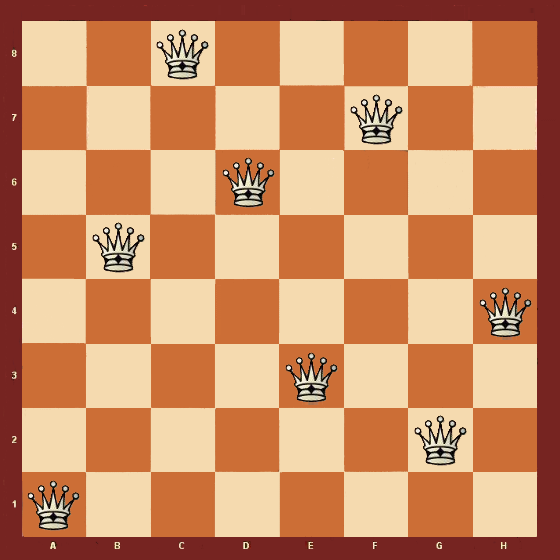# 八皇后问题怎么解决？

八皇后的解决办法有很多种，我们这里采取回溯法解决。

## 什么是回溯法

回溯法的处理思想类似于枚举搜索，我们枚举出每一种情况，然后在根据条件进行筛选，找到满足期望的值。我们把求解过程分为多个阶段，每个阶段我们都会面临一个十字路口，我们随便找一条路，走不通后，就回到上一个十字路口，选择另一个十字路口进行下一步，知道遍历完整个枚举情况。

## 回溯法的模板

在面临较简单的回溯问题是可以使用以下模板

void backtracking(参数) {
if (终止条件) {
存放结果;
return;
}

for (选择：本层集合中元素（树中节点孩子的数量就是集合的大小）) {
处理节点;
backtracking(路径，选择列表); // 递归
回溯，撤销处理结果
}
}

# 八皇后问题的核心代码

int []Queen=new int;//存取皇后所在列的位置
public void eightQueen(int row){

if (row==8){//当row等于8时说明八个皇后都放置好了
printQueens(Queen);//打印八皇后
return;
}
for (int column = 0; column < 8; column++) {
if (isOk(row,column)){//这里判断是否可以放在这个位置
Queen[row]=column;//放置皇后
eightQueen(row+1);
}
}
}

那么有小伙伴就迷惑了，八皇后不应该是八行八列吗，为什么数组只是一个一维数组。这里就用到了回溯法的思想，把八行八列分成了八行，每一行都独立，而一维数组则只用来存取皇后的列坐标，行坐标由数组的下标代替。

## 判断皇后位置是否可行

public boolean isOk(int row,int column){
int leftup=column-1,rightup=column+1;
for (int i = row-1; i >=0 ; --i) {
if (Queen[i]==column) return false;//看垂直方向是否有皇后
if (leftup>=0){
if (Queen[i]==leftup) return false;//看左上角斜线是否有皇后
}
if (rightup<8){
if (Queen[i]==rightup) return false;//看右上角斜线是否有皇后
}
--leftup;
++rightup;
}
return true;
}

判断位置可行的条件是，是否存在有皇后处在同一列，同一行（同一行的情况不存在，因为row一直加1），同一斜线。

那么判断同一列我们只要看一维数组中是否有值与将要放置的皇后的列一致，如果一致我们为判断false;

那么如何判断是否在同一条斜线，

假设一个点A AA的坐标是[ a , b ] [a,b][a,b]，那么和该点在同一斜线上的点A 有四种,分别是
[ a + x , b + x ] 、 [ a − x , b − x ] 、 [ a + x , b − x ] 、 [ a − x , b + x ]

而我们只考虑左右上角的位置是否存在同一条斜线,那么为什么不考虑下角是否存在呢，因为我们的皇后还没排到左右下角，所以只考虑 [ a − x , b + x ] 和[ a − x , b − x ]这两种情况。

我们通过让x等于1时来达到，当处于同一斜线时，斜线分为四十五度，则行和列相等时，在同一斜线上，判断为false.

# 八皇后总体实现代码

public static void main(String[] args) {
Solution1 solution=new Solution1();
solution.eightQueen(0);
}
int []Queen=new int;
int count=0;
public void eightQueen(int row){

if (row==8){
count++;
printQueens(Queen);
return;
}
for (int column = 0; column < 8; column++) {
if (isOk(row,column)){

Queen[row]=column;
eightQueen(row+1);
}
}
}
public boolean isOk(int row,int column){
int leftup=column-1,rightup=column+1;
for (int i = row-1; i >=0 ; --i) {
if (Queen[i]==column) return false;//看垂直方向是否有皇后
if (leftup>=0){
if (Queen[i]==leftup) return false;//看左上角斜线是否有皇后
}
if (rightup<8){
if (Queen[i]==rightup) return false;//看右上角斜线是否有皇后
}
--leftup;
++rightup;
}
return true;
}
private void printQueens(int []Queen){
for (int row = 0; row < 8; row++) {
for (int column = 0; column < 8; column++) {
if (Queen[row]==column) System.out.print("   Q   ");
else System.out.print("   *   ");
}
System.out.println();
}
System.out.print(count);
System.out.println();
}
}

# 种一棵树的最好时间是十年前，其次是现在。

展开全文java 算法 数据结构 推荐算法
• 以4皇后为例，其他的N皇后问题以此类推。所谓4皇后问题就是求解如何在4×4的棋盘上无冲突的摆放4个皇后棋子。在国际象棋中，皇后的移动方式为横竖交叉的，因此在任意一个皇后所在位置的水平、竖直、以及45度斜线上都...
• 回溯法，又被称为“试探法”。解决问题时，每进行一步，都是抱着试试看的态度，如果发现当前选择并不是最好的，或者这么走下去肯定达不到目标，立刻做回退操作... 回溯法问题本身出发，寻找可能实现的所有情况...

回溯法，又被称为“试探法”。解决问题时，每进行一步，都是抱着试试看的态度，如果发现当前选择并不是最好的，或者这么走下去肯定达不到目标，立刻做回退操作重新选择。这种走不通就回退再走的方法就是回溯法。

## 回溯VS递归

很多人认为回溯和递归是一样的，其实不然。在回溯法中可以看到有递归的身影，但是两者是有区别的。

回溯法从问题本身出发，寻找可能实现的所有情况。和穷举法的思想相近，不同在于穷举法是将所有的情况都列举出来以后再一一筛选，而回溯法在列举过程如果发现当前情况根本不可能存在，就停止后续的所有工作，返回上一步进行新的尝试。

递归是从问题的结果出发，例如求 n！，要想知道 n！的结果，就需要知道 n*(n-1)! 的结果，而要想知道 (n-1)! 结果，就需要提前知道 (n-1)*(n-2)!。这样不断地向自己提问，不断地调用自己的思想就是递归。

回溯和递归唯一的联系就是，回溯法可以用递归思想实现。

## 回溯法与树的遍历

使用回溯法解决问题的过程，实际上是建立一棵“状态树”的过程。例如，在解决列举集合{1,2,3}所有子集的问题中，对于每个元素，都有两种状态，取还是舍，所以构建的状态树为：图1 状态树

回溯法的求解过程实质上是先序遍历“状态树”的过程。树中每一个叶子结点，都有可能是问题的答案。图 1 中的状态树是满二叉树，得到的叶子结点全部都是问题的解。

在某些情况下，回溯法解决问题的过程中创建的状态树并不都是满二叉树，因为在试探的过程中，有时会发现此种情况下，再往下进行没有意义，所以会放弃这条死路，回溯到上一步。在树中的体现，就是在树的最后一层不是满的，即不是满二叉树，需要自己判断哪些叶子结点代表的是正确的结果。

## 回溯法解决八皇后问题

八皇后问题是以国际象棋为背景的问题：有八个皇后（可以当成八个棋子），如何在 8*8 的棋盘中放置八个皇后，使得任意两个皇后都不在同一条横线、纵线或者斜线上。图 2 八皇后问题示例（#代表皇后）

八皇后问题是使用回溯法解决的典型案例。算法的解决思路是：

1. 从棋盘的第一行开始，从第一个位置开始，依次判断当前位置是否能够放置皇后，判断的依据为：同该行之前的所有行中皇后的所在位置进行比较，如果在同一列，或者在同一条斜线上（斜线有两条，为正方形的两个对角线），都不符合要求，继续检验后序的位置。
2. 如果该行所有位置都不符合要求，则回溯到前一行，改变皇后的位置，继续试探。
3. 如果试探到最后一行，所有皇后摆放完毕，则直接打印出 8*8 的棋盘。最后一定要记得将棋盘恢复原样，避免影响下一次摆放。

源代码：

#include <stdio.h>
int Queenes={0},Counts=0;
int Check(int line,int list){
//遍历该行之前的所有行
for (int index=0; index<line; index++) {
//挨个取出前面行中皇后所在位置的列坐标
int data=Queenes[index];
//如果在同一列，该位置不能放
if (list==data) {
return 0;
}
//如果当前位置的斜上方有皇后，在一条斜线上，也不行
if ((index+data)==(line+list)) {
return 0;
}
//如果当前位置的斜下方有皇后，在一条斜线上，也不行
if ((index-data)==(line-list)) {
return 0;
}
}
//如果以上情况都不是，当前位置就可以放皇后
return 1;
}
//输出语句
void print()
{
for (int line = 0; line < 8; line++)
{
int list;
for (list = 0; list < Queenes[line]; list++)
printf("0");
printf("#");
for (list = Queenes[line] + 1; list < 8; list++){
printf("0");
}
printf("\n");
}
printf("================\n");
}
void eight_queen(int line){
//在数组中为0-7列
for (int list=0; list<8; list++) {
//对于固定的行列，检查是否和之前的皇后位置冲突
if (Check(line, list)) {
//不冲突，以行为下标的数组位置记录列数
Queenes[line]=list;
//如果最后一样也不冲突，证明为一个正确的摆法
if (line==7) {
//统计摆法的Counts加1
Counts++;
//输出这个摆法
print();
//每次成功，都要将数组重归为0
Queenes[line]=0;
return;
}
//继续判断下一样皇后的摆法，递归
eight_queen(line+1);
//不管成功失败，该位置都要重新归0，以便重复使用。
Queenes[line]=0;
}
}
}
int main() {
//调用回溯函数，参数0表示从棋盘的第一行开始判断
eight_queen(0);
printf("摆放的方式有%d种",Counts);
return 0;
}

### 因为八皇后摆放方式有92种，这里也不再一一列举。

下面的是个人的一点解决方法，算不上完全解决了这个问题，说到底还是回溯法没有用好，只能输出一种解决方法。

源代码：

int queens = { 0 };
int count = 0;
//check函数，若通过检查返回1，否则返回0
int check(int x, int y)
{
for (int i = 0; i < x; i++)
{
//遍历之前所有行，若有重复 则返回0
if (queens[i] == y)
return 0;
}

for (int i = 0; i < x; i++)
{
int qx, qy;
qx = i;
qy = queens[i];
if (abs(x - qx) == abs(y - qy))
return 0;
}
return 1;
}
//goback,如果发现这一层任何位置都不可以放置皇后，则回退到上一层
void goback()
{
count--;

if (queens[count] == 7)
goback();
else
queens[count] =queens[count]++;
while (check(count, queens[count]) == 0)
{
if (queens[count] == 7)
goback();
else
queens[count]++;
}

}
//显示8皇后位置
void showPos()
{
countShow++;
for (int i = 0; i < 8; i++)
{
for (int j = 0; j < 8; j++)
{
if (queens[i] == j)
printf("1");
else
printf("0");
}
printf("\n");
}
for (int i = 0; i < 8; i++)
{
printf("%d ", queens[i]);
}
printf("第%d个八皇后\n",countShow);
}
int main()
{
while (count!=8)
{
for (int i = 0; i < 8; i++)
{
queens[count] = i;
if (check(count, i))
break;
if (i == 7)
goback();
}
count++;
}
showPos();
system("pause");
return 0;
}

展开全文• 主要介绍了C语言基于回溯算法解决八皇后问题的方法,简单描述了八皇后问题,并结合实例形式分析了C语言使用回溯算法解决八皇后问题的相关操作技巧,需要的朋友可以参考下
• javascript 递归回溯法解八皇后问题 网上看到许多关于八皇后算法的文章 很少能看到使用 javascript 来实现的 今天就给 大家使用 javascript 来解决下这个问题有需要的小伙伴可以参考下 下面给大家分享的是回溯法解八...
• 课程的随堂作业，C语言的，用dev就能运行，萌新代码，勿喷，仅仅帮助不想写作业的朋友方便一下，反正老师也不会仔细检查的C语言
• 程序分析：在8*8的棋盘上安置八个皇后且相互不能攻击。 常见解法有： 蛮力搜索 一个皇后的攻击范围如下图所示： 其中一种摆如下：视频教学链接 ：https://www.51zxw.net/Show.aspx?cid=717&id=79065。 ...

程序分析：在8*8的棋盘上安置八个皇后且相互不能攻击。

一个皇后的攻击范围如下图所示：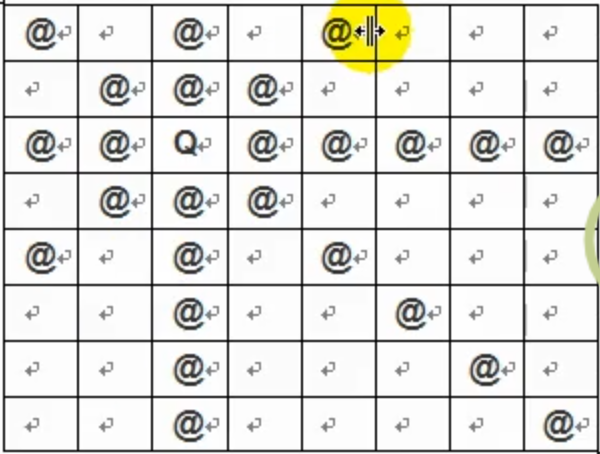其中一种摆法如下：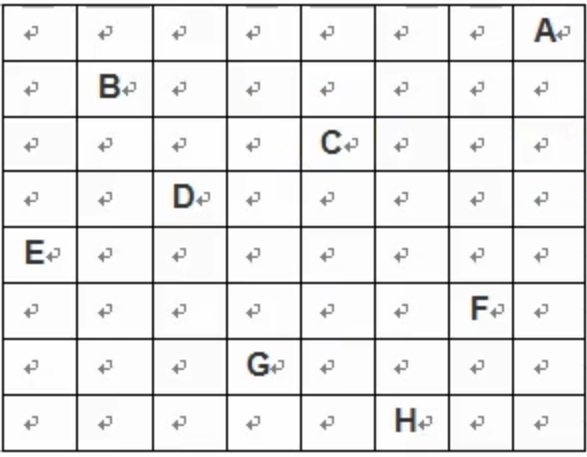C++代码：

方法一：

#include <iostream>
using namespace std;

const int ArSize = 8;//这个数等于几，就是几皇后。
int num = 0;

bool check(bool arr[ArSize][ArSize], int row, int column){
// 判断皇后的落点是否合规
if (row == 0){
return true;
}
int i, j;

for (i = 0; i < row; ++i){
// 判断纵向是否有冲突
if (arr[i][column]){
return false;
}
}

i = row - 1;
j = column - 1;
while (i >= 0 && j >= 0){
// 判断正斜对角线是否有冲突
if (arr[i][j]){
return false;
}
--i;
--j;
}

i = row - 1;
j = column + 1;
while (i >= 0 && j <= ArSize - 1){
// 判断负斜对角线是否有冲突
if (arr[i][j]){
return false;
}
--i;
++j;
}
return true;
}

void outPut(bool arr[ArSize][ArSize]){
// 打印每种正确的解法
++num;
cout << "**********************" << num << "*********************" << endl;
for (int i = 0; i < ArSize; ++i){
for (int j = 0; j < ArSize; ++j){
cout << arr[i][j] << " ";
}
cout << endl;
}
cout << "*********************************************" << endl;
}

void solve(bool arr[ArSize][ArSize], int row) {
// 回溯法
for (int column = 0; column < ArSize; ++column) {
arr[row][column] = true;
if (check(arr, row, column)) {
if (row + 1 == ArSize) {
outPut(arr);
}
else {
solve(arr, row + 1);
}
}
arr[row][column] = false;
}
}

int main()
{
bool chessboard[ArSize][ArSize];
// 数组初始化
for (auto &i : chessboard){
for (auto &j : i){
j = false;
}
}
/*
//数组初始化方法二
for (int i = 0; i < ArSize; i++) {
for (int j = 0; j < ArSize; j++) {
chessboard[i][j] = false;
}
}
*/
solve(chessboard, 0);
cout << "八皇后问题共有" << num << "种解！" << endl;
system("pause");
return 0;
}


方法二：

#include<iostream>
#include<vector>//用向量保存皇后
using namespace std;

class Queen {
public:
short x;
short y;
Queen(short _x, short _y) :x(_x), y(_y) {}
bool Attack(Queen &queen) {
//能相互攻击返回true
return x == queen.x || x - y == queen.x - queen.y || x + y == queen.x + queen.y || y == queen.y;
}
};

void PrintQueens(vector<Queen> &v,int *count) {
cout << "第" << *count << "种摆法：" << endl;
short i, j;
vector<Queen>::iterator itr1 = v.begin();
for (i = 0; i < 8; i++) {
for (j = 0; j < 8; j++) {
if (itr1 != v.end()&&i == itr1->y&&j == itr1->x) {
cout << "后";
itr1++;
}
else {
cout << "□";
}

}
cout << endl;
}
(*count)++;
}

int main() {
vector<Queen> vt;
short i, j;
vector<Queen>::iterator itr;//迭代器
Queen *q;
int count=1;
for (i = 0; i < 8; i++) {
j = 0;
Repeat:
for (; j < 8; j++) {
q = new Queen(j, i);
for (itr = vt.begin(); itr != vt.end(); itr++) {
if (q->Attack(*itr)) {
//新皇后与原来的皇后进行攻击测试
delete q;
break;
}
}
if (itr == vt.end()) {
//新皇后与原来皇后不冲突
vt.push_back(*q);
delete q;
if (vt.size() == 8) {
PrintQueens(vt, &count);//输出八皇后解法
j = 8;//人为赋值，使流程进入 if（j==8）回溯重试
}
break;//退出列遍历，直接到下一行的第一列
}
}
if (j == 8) {
//当前的一整行都无法安放皇后
if (vt.back().x == 7) {
//向量尾部的皇后若刚好是当前行最后一列的位置，不能将x后移，必须多弹出一次
vt.pop_back();//弹出
}
if (!vt.empty()) {
i = vt.back().y;
j = vt.back().x + 1;
vt.pop_back();
goto Repeat;
}
}
}
return 0;
}


C++运行结果：

方法一：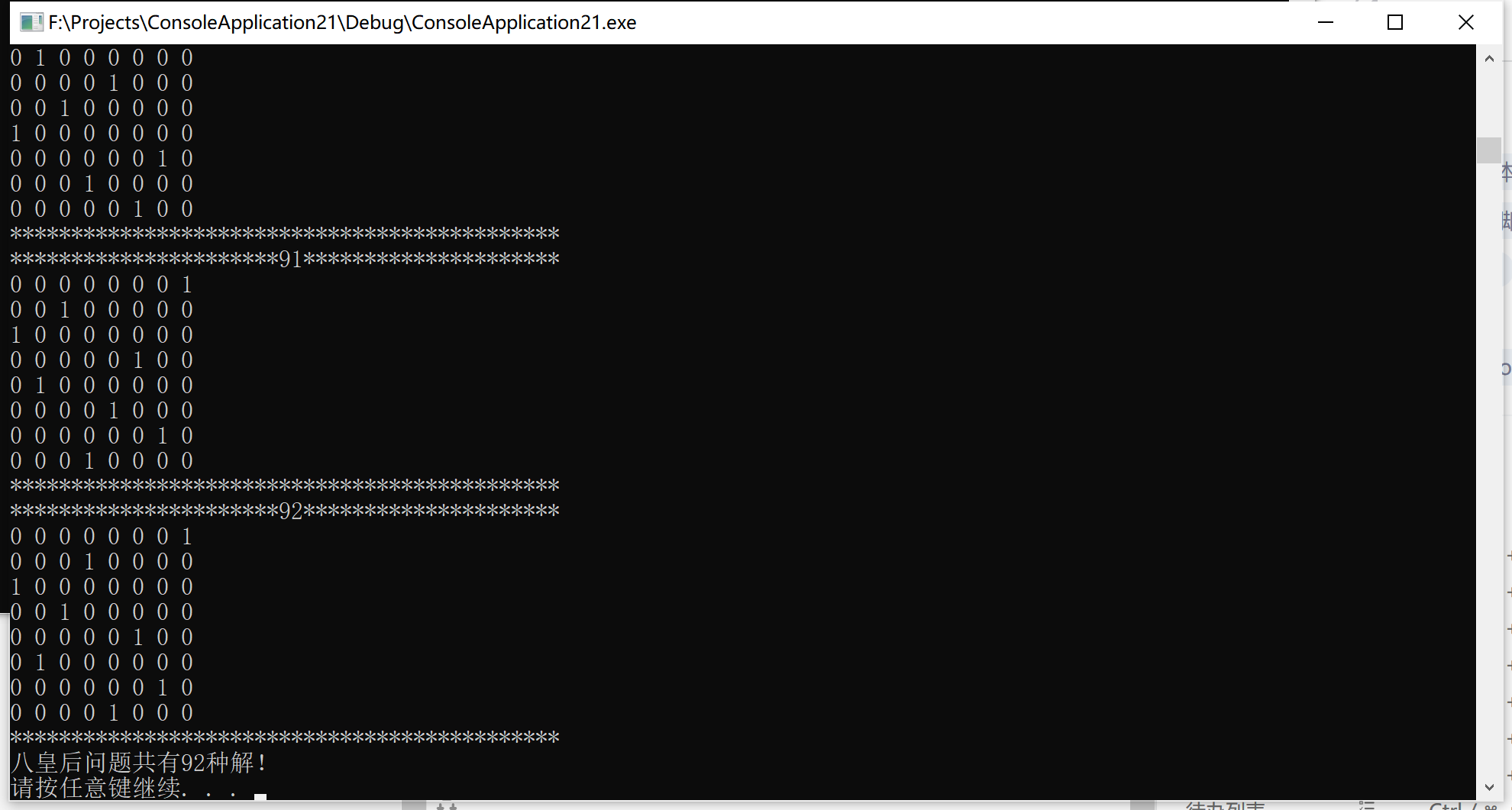方法二：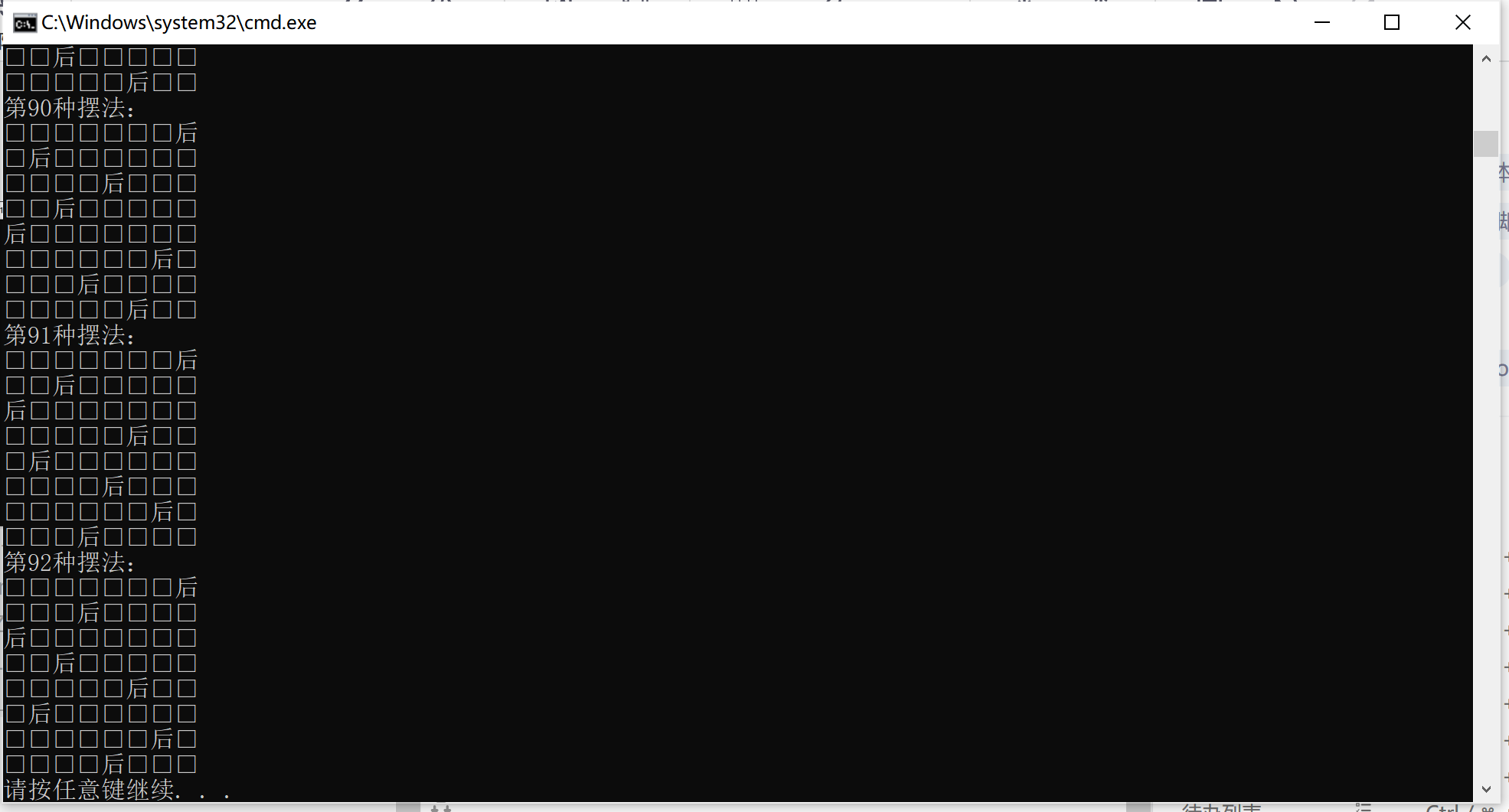展开全文c++
• 皇后问题：在8×8格的国际象棋上摆放八个皇后，任意两个皇后都不能处于同一行、同一列或同一斜线上，问有多少种摆。 将棋盘抽象为4 * 4到20 * 20大小的矩阵 输入样例: 请输入棋盘的大小（4 ~ 20）： 4 ...c语言 算法
• 回溯法 * @author zc * */ public class Queen8 { int max = 8; int[] arrs = new int[max]; static int count = 0; public static void main(String[] args) { Queen8 queen8 = new Queen8(); queen8...
• 皇后问题（递归回溯法问题 在一个8*8的棋盘中，有八个皇后的棋子。这些棋子所放的位置的同一行，同一列和同一个斜线上不能出现另一个皇后，问有多少种摆放的方式。 思路 （1）先将一个皇后放到第一行的第一列 ...java 算法
• 皇后是经典的回溯法问题，题目是说将八个皇后，放到8×8的国际象棋棋盘中中，使得任意两个皇后都不能在同一行、同一列以及同一条对角线上。这个问题可以通过暴力法求解，代码也很短： for(solu = 1; solu &...
• 皇后问题是一个古老的问题，是回溯算法的典型案例。该问题是国际西洋棋手马克思.贝瑟尔于1848年提出:在8*8格子的国际象棋上摆放八个皇后，使其不能互相攻击，任意两个皇后不能在一列，不能在一条斜线上。（92种） ...算法 数据结构
•c++
• 皇后问题是典型的回溯法的应用，而回溯法的本质就是树的遍历和剪枝，N皇后问题可以说是N叉树的遍历和剪枝问题。对于树的遍历问题都是能抽象出解题模板的，见后续分析。完整代码在文章末尾。这里主要详细记录一下...算法 python
• ## n皇后问题(回溯法)

千次阅读 2022-05-10 19:03:05
问题是:在8×8的棋盘上摆放八个皇后，使其不能互相攻击，即任意两个皇后都不能处于同一行、同一列或同一斜线上。可以把八皇后问题扩展到n皇后问题，即在n×n的棋盘上摆放n个皇后，使任意两个皇后都不能处于同一行、...算法 c++ 逻辑回归 深度优先
• 问题表述为:在8×8格的国际象棋上摆放8皇后，使其不能互相攻击，即任意两个皇后都不能处于同一行、同一列或同一斜线上，问有多少种摆。高斯认为有76种方案。1854年在柏林的象棋杂志上不同的作者发表了40种不同的...算法
• 主要介绍了Python基于回溯法子集树模板实现8皇后问题,简单说明了8皇后问题的原理并结合实例形式分析了Python回溯法子集树模板解决8皇后问题的具体实现技巧,需要的朋友可以参考下
• 采用的是利用递归进行回溯法 题目： 在NN的方格棋盘放置了N个皇后，使得它们不相互攻击（即任意2个皇后不允许处在同一排，同一列，也不允许处在与棋盘边框成45角的斜线上。 你的任务是，对于给定的N，求出有多少种...算法 深度优先 数据结构
• 下面给大家分享的是回溯法解八皇后, 带详细注解，这里就不多废话了。 function NQueens(order) { if (order < 4) { console.log('N Queens problem apply for order bigger than 3 ! '); return; } var n...
• 主要介绍了C语言八皇后问题解决方法,简单描述了八皇后问题并结合实例形式分析了C语言基于暴力法与回溯法解决八皇后的具体操作技巧,需要的朋友可以参考下
• 读完本文这个小故事，相信你会完全弄懂N皇后问题。 故事还得从中国象棋说起… 文章目录中国象棋 — 国王背后的女人国王的烦恼 — 安排皇后国王的后宫 — N皇后一句话概括N皇后问题 中国象棋 — 国王背后的女人 中国...
• 问题描述 　在N*N的方格棋盘放置了N个皇后，使得它们不相互攻击（即任意2个皇后不允许处在同一排，同一列，也不允许处在与棋盘边框成45角的斜线上。你的任务是，对于给定的N，求出有多少种合法的放置方法。 输入...动态规划 算法 c++
• N皇后问题回溯法、FIFO分支限界算法，内部包含两个函数，在main函数中分别运行。...